Courses

# Cables Mechanical Engineering Notes | EduRev

## Mechanical Engineering : Cables Mechanical Engineering Notes | EduRev

The document Cables Mechanical Engineering Notes | EduRev is a part of the Mechanical Engineering Course Engineering Mechanics - Notes, Videos, MCQs & PPTs.
All you need of Mechanical Engineering at this link: Mechanical Engineering

Introduction

Cables and arches are closely related to each other and hence they are grouped in this course in the same module. For long span structures (for e.g. in case bridges) engineers commonly use cable or arch construction due to their efficiency. In the first lesson of this module, cables subjected to uniform and concentrated loads are discussed. In the second lesson, arches in general and three hinged arches in particular along with illustrative examples are explained. In the last two lessons of this module, two hinged arch and hingeless arches are considered.

Structure may be classified into rigid and deformable structures depending on change in geometry of the structure while supporting the load. Rigid structures support externally applied loads without appreciable change in their shape (geometry). Beams trusses and frames are examples of rigid structures. Unlike rigid structures, deformable structures undergo changes in their shape according to externally applied loads. However, it should be noted that deformations are still small. Cables and fabric structures are deformable structures. Cables are mainly used to support suspension roofs, bridges and cable car system. They are also used in electrical transmission lines and for structures supporting radio antennas. In the following sections, cables subjected to concentrated load and cables subjected to uniform loads are considered.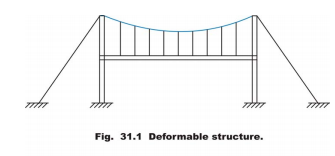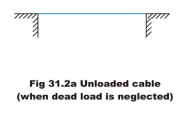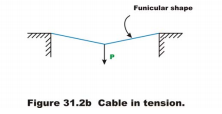The shape assumed by a rope or a chain (with no stiffness) under the action of external loads when hung from two supports is known as a funicular shape. Cable is a funicular structure. It is easy to visualize that a cable hung from two supports subjected to external load must be in tension (vide Fig. 31.2a and 31.2b). Now let us modify our definition of cable. A cable may be defined as the structure in pure tension having the funicular shape of the load.

As stated earlier, the cables are considered to be perfectly flexible (no flexural stiffness) and inextensible. As they are flexible they do not resist shear force and bending moment. It is subjected to axial tension only and it is always acting tangential to the cable at any point along the length. If the weight of the cable is negligible as compared with the externally applied loads then its self weight is neglected in the analysis. In the present analysis self weight is not considered.

Consider a cable as loaded in Fig. 31.2. Let us assume that the cable lengths L1,L2,L3,L4 and sag at C,D,E(hc,hd,he ) are known. The four reaction components at A and B , cable tensions in each of the four segments and three sag values: a total of eleven unknown quantities are to be determined. From the geometry, one could write two force equilibrium equations (∑Fx = 0,∑Fy=0 ) at each of the point A,B,C,D  and E i.e. a total of ten equations and the required one more equation may be written from the geometry of the cable. For example, if one of the sag is given then the problem can be solved easily. Otherwise if the total length of the cable S is given then the required equation may be written as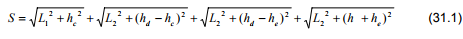Cables are used to support the dead weight and live loads of the bridge decks having long spans. The bridge decks are suspended from the cable using the hangers. The stiffened deck prevents the supporting cable from changing its shape by distributing the live load moving over it, for a longer length of cable. In such cases cable is assumed to be uniformly loaded.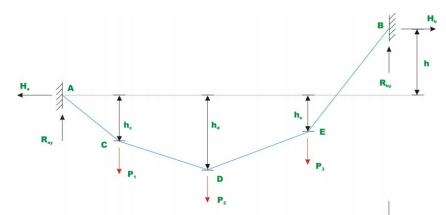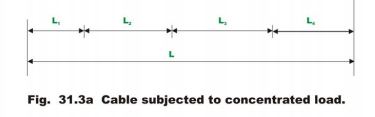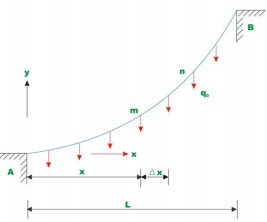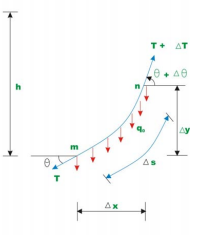Fig. 31.3b Cable subjected to uniformly Fig. 31.3c Free-body diagram distributed load.

Consider a cable which is uniformly loaded as shown in Fig 31.3a. Let the slope of the cable be zero at A . Let us determine the shape of the cable subjected to uniformly distributed load q0. Consider a free body diagram of the cable as shown in Fig 31.3b. As the cable is uniformly loaded, the tension in the cable changes continuously along the cable length. Let the tension in the cable at m end of the free body diagram be T and tension at the  end of the cable be T + TΔ. The slopes of the cable at  and  are denoted by θ and θ +Δθ respectively. Applying equations of equilibrium, we get

∑Fy =0      −T sinθ + (T + ΔT ) sin(θ + Δθ ) − q0 (Δx) = 0       (31 .2a)

∑Fx = 0     −T cosθ + (T + ΔT ) cos(θ + Δθ ) = 0                    (31.2b )

∑Mn = 0    − (T cosθ)Δy +(Tsinθ)Δx+(q0+Δx)Δx/2 = 0           (31.2 c)

Dividing equations 31.2a, b, c by Δx and noting that in the limit as Δx → 0, Δy → 0 Δθ → 0 and ΔT → 0 .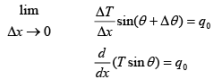(31.3a)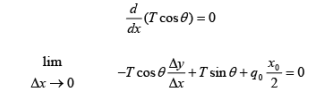(31  . 3b)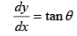(31.3c)

Integrating equation (31.3b) we get

T cosθ = constant

At support (i.e., at x = 0 ),    T cosθ = H  (31.4a)

i.e. horizontal component of the force along the length of the cable is constant. Integrating equation 31.3a,

T sinθ = q0x + C1

At x = 0, T sinθ = 0, C1 = 0          as θ = 0 at that point.

Hence, T sinθ = q0 x                 (31.4b)

From equations 31.4a and 31.4b, one could write

tanθ = q0x/H            (31.4c)

From the figure,   tanθ =dy/dx =q0x/H

∴ y =q0x2/2H +C

At x =0, y= 0 ⇒C=0 and y=q0x2/2H       (31.5)

Equation 31.5 represents a parabola. Now the tension in the cable may be evaluated from equations 31.4a and 31.4b. i.e,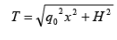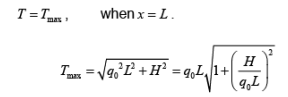(31.6)

Due to uniformly distributed load, the cable takes a parabolic shape. However due to its own dead weight it takes a shape of a catenary. However dead weight of the cable is neglected in the present analysis.

Offer running on EduRev: Apply code STAYHOME200 to get INR 200 off on our premium plan EduRev Infinity!

,

,

,

,

,

,

,

,

,

,

,

,

,

,

,

,

,

,

,

,

,

;